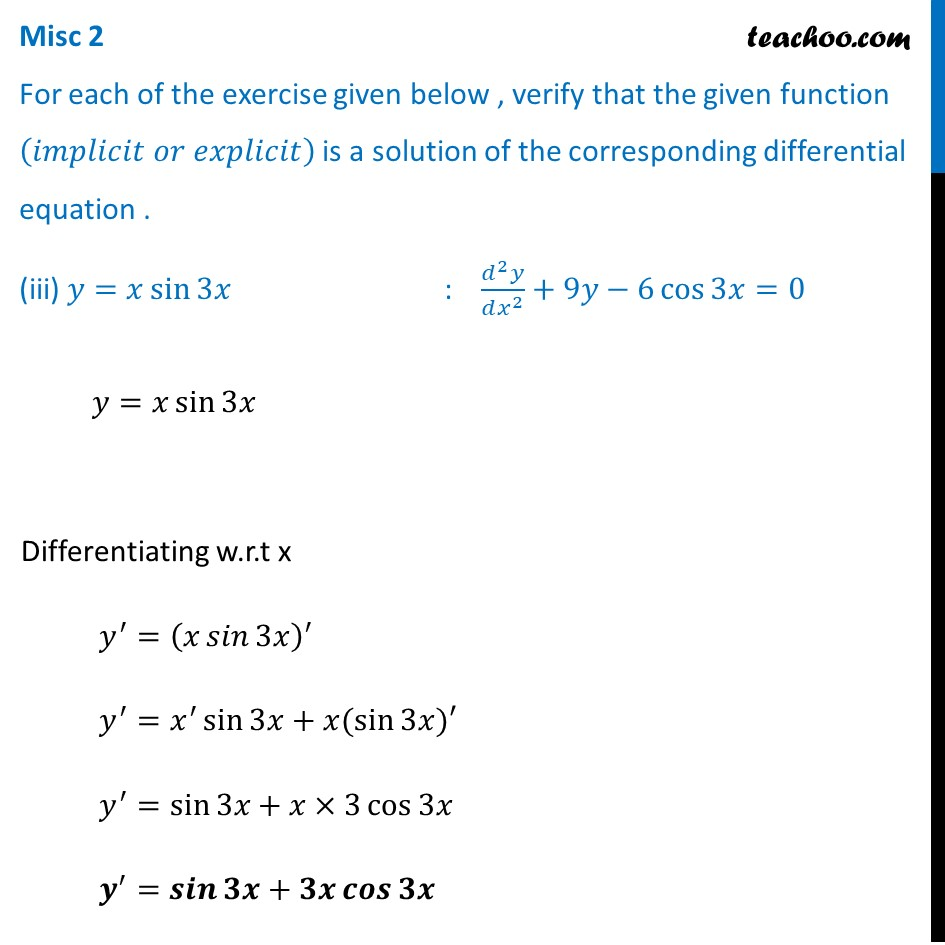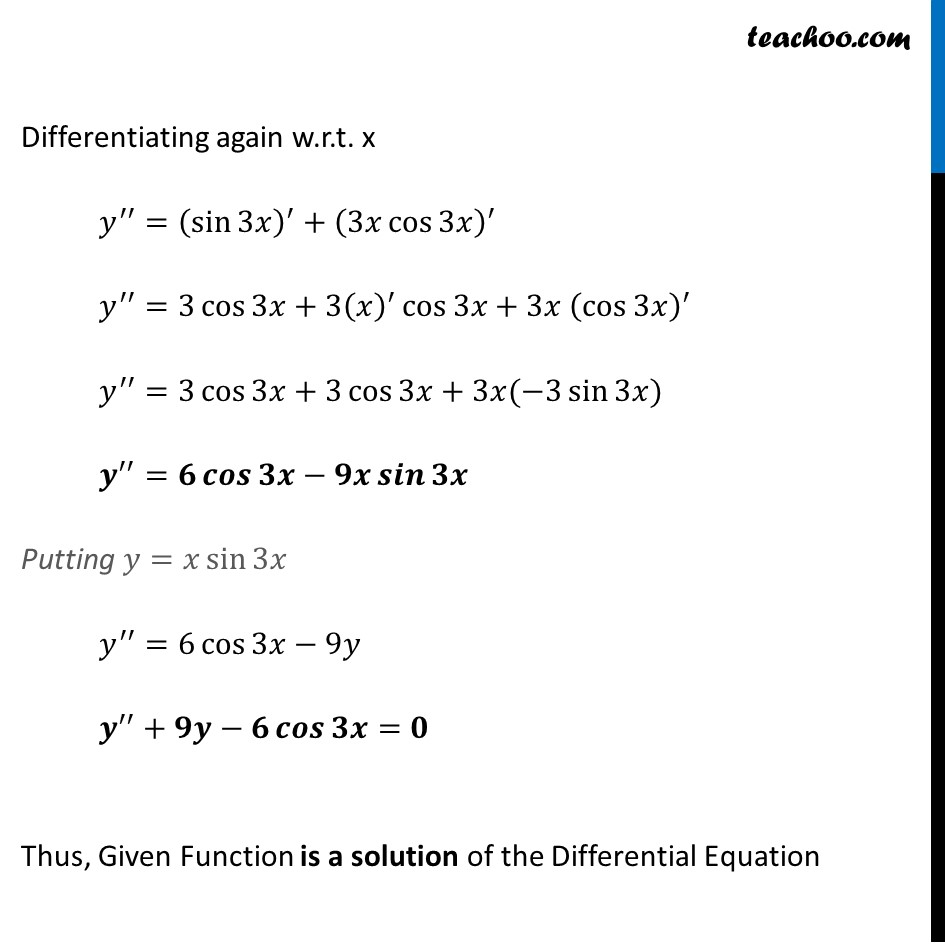Miscellaneous

Chapter 9 Class 12 Differential Equations
Serial order wiseLearn in your speed, with individual attention - Teachoo Maths 1-on-1 Class

### Transcript

Misc 2 For each of the exercise given below , verify that the given function (𝑖𝑚𝑝𝑙𝑖𝑐𝑖𝑡 𝑜𝑟 𝑒𝑥𝑝𝑙𝑖𝑐𝑖𝑡) is a solution of the corresponding differential equation . (iii) 𝑦=𝑥 sin⁡3𝑥 : (𝑑^2 𝑦)/(𝑑𝑥^2 )+9𝑦−6 cos⁡〖3𝑥=0〗 𝑦=𝑥 sin⁡3𝑥 Differentiating w.r.t x 𝑦^′=(𝑥 𝑠𝑖𝑛⁡3𝑥 )^′ 𝑦^′=𝑥^′ sin⁡3𝑥+𝑥(sin⁡3𝑥)′ 𝑦^′=sin⁡3𝑥+𝑥×3 cos⁡3𝑥 𝒚^′=𝒔𝒊𝒏⁡𝟑𝒙+𝟑𝒙 𝒄𝒐𝒔⁡𝟑𝒙 Differentiating again w.r.t. x 𝑦^′′=(sin⁡3𝑥 )^′+(3𝑥 cos⁡3𝑥 )^′ 𝑦^′′=3 cos⁡3𝑥+〖3(𝑥)〗^′ cos⁡3𝑥+3𝑥 (cos⁡3𝑥 )^′ 𝑦^′′=3 cos⁡3𝑥+3 cos⁡3𝑥+3𝑥(−3 sin⁡3𝑥) 𝒚^′′=𝟔 𝒄𝒐𝒔⁡𝟑𝒙−𝟗𝒙 𝒔𝒊𝒏⁡𝟑𝒙 Putting 𝑦=𝑥 sin⁡3𝑥 𝑦^′′=6 cos⁡3𝑥−9𝑦 𝒚^′′+𝟗𝒚−𝟔 𝒄𝒐𝒔⁡𝟑𝒙=𝟎 Thus, Given Function is a solution of the Differential Equation